# Weight¶

You can change the shape of the desirability for each goal by the “weight” field. Use weights to give added emphasis to the upper/lower bounds, or to emphasize the target value. With a weight of 1 the di will vary from 0 to 1 in a linear fashion. Weights greater than 1 (maximum weight is 10), give more emphasis to the goal. Weights less than 1 (minimum weight is 0.1), give less emphasis to the goal. The formulas and outcomes for various options can be seen below. These shapes will be displayed by Design-Expert. You can drag the curves with your mouse and change their shape.

For goal of maximum, the desirability will be defined by the following formulas: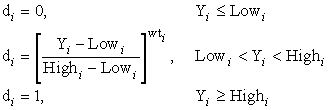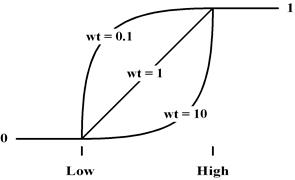Desirability Curves for Goal is Maximum

For goal of minimum, the desirability will be defined by the following formulas: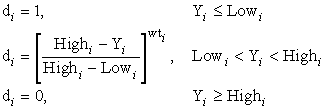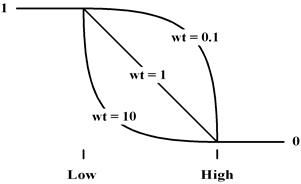Desirability Curves for Goal is Minimum

For goal as a target, the desirability will be defined by the following formulas: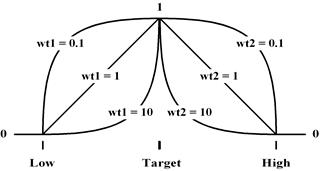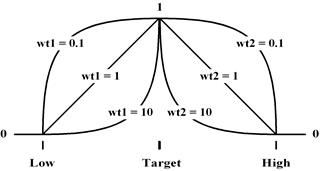Desirability Curves for Goal is Target

For goal within range (a constraint), desirability will be defined by the following formulas: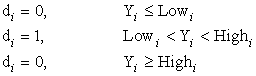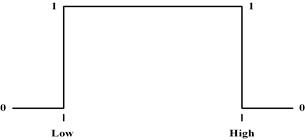Desirability Curves for Goal as Range

Within the program, we modify the desirability function by adding a “tail” as the function evaluation approaches zero. The tail adjustment is reduced with each iteration to drive the function towards its pure value. Otherwise the optimization would get stuck on the zero desirability planes.